基本形式

$f(x)=w_1x_1+w_2x_2+...+w_dx_d+b$

$f(x)=w^Tx+b$

$h_\theta(x)=\theta_0x_0+\theta_1x_1+...+\theta_nx_n=\theta^Tx$
$\theta_0$为截距，在sklearn中为intercept_

Cost function

定义

$J(\theta)=\sum_{i=1}^m(h_\theta(x^{(i)})-y^{(i)})^2$

岭回归（Ridge）

$J(\theta)=\sum_{i=1}^m(h_\theta(x^{(i)})-y^{(i)})^2+\alpha\sum_{j=0}^n\theta_j^2$

Lasso

$J(\theta)=\frac{1}{2m}\sum_{i=1}^m(h_\theta(x^{(i)})-y^{(i)})^2+\alpha\sum_{j=0}^n|\theta_j|$

弹性网络

$J(\theta)=\frac{1}{2m}\sum_{i=1}^m(h_\theta(x^{(i)})-y^{(i)})^2+\alpha\rho\sum_{j=0}^n|\theta_j|+\frac{\alpha(1-\rho)}{2}\sum_{j=0}^n\theta_j^2$

由来（概率解释）

$y^{(i)}=\theta^Tx^{(i)}+\epsilon^{(i)}$

$p(\epsilon^{(i)}) = \frac{1}{\sqrt{2\pi}\sigma}\exp(-\frac{(\epsilon^{(i)})^2}{2\sigma^2})\\ p(y^{(i)}|x^{(i)};\theta) = \frac{1}{\sqrt{2\pi}\sigma}\exp(-\frac{(y^{(i)} - \theta^Tx^{(i)})^2}{2\sigma^2})$

$L(\theta) =\prod_{i=1}^{m} p(y^{(i)}|x^{(i)};\theta) =\prod_{i=1}^{m}\frac{1}{\sqrt{2\pi}\sigma}\exp(-\frac{(y^{(i)} - \theta^Tx^{(i)})^2}{2\sigma^2})$

$log\ L(\theta) =log\ \prod_{i=1}^{m}\frac{1}{\sqrt{2\pi}\sigma}\exp(-\frac{(y^{(i)} - \theta^Tx^{(i)})^2}{2\sigma^2})\\ log\ L(\theta)=\sum_{i=1}^{m}log\ \frac{1}{\sqrt{2\pi}\sigma}-\frac{1}{\sigma^2}\cdot\frac{1}{2}\sum_{i=1}^{m}(y^{(i)} - \theta^Tx^{(i)})^2$

$\frac{1}{2}\sum_{i=1}^{m}(y^{(i)} - \theta^Tx^{(i)})^2$

求解

普通最小二乘法

正规方程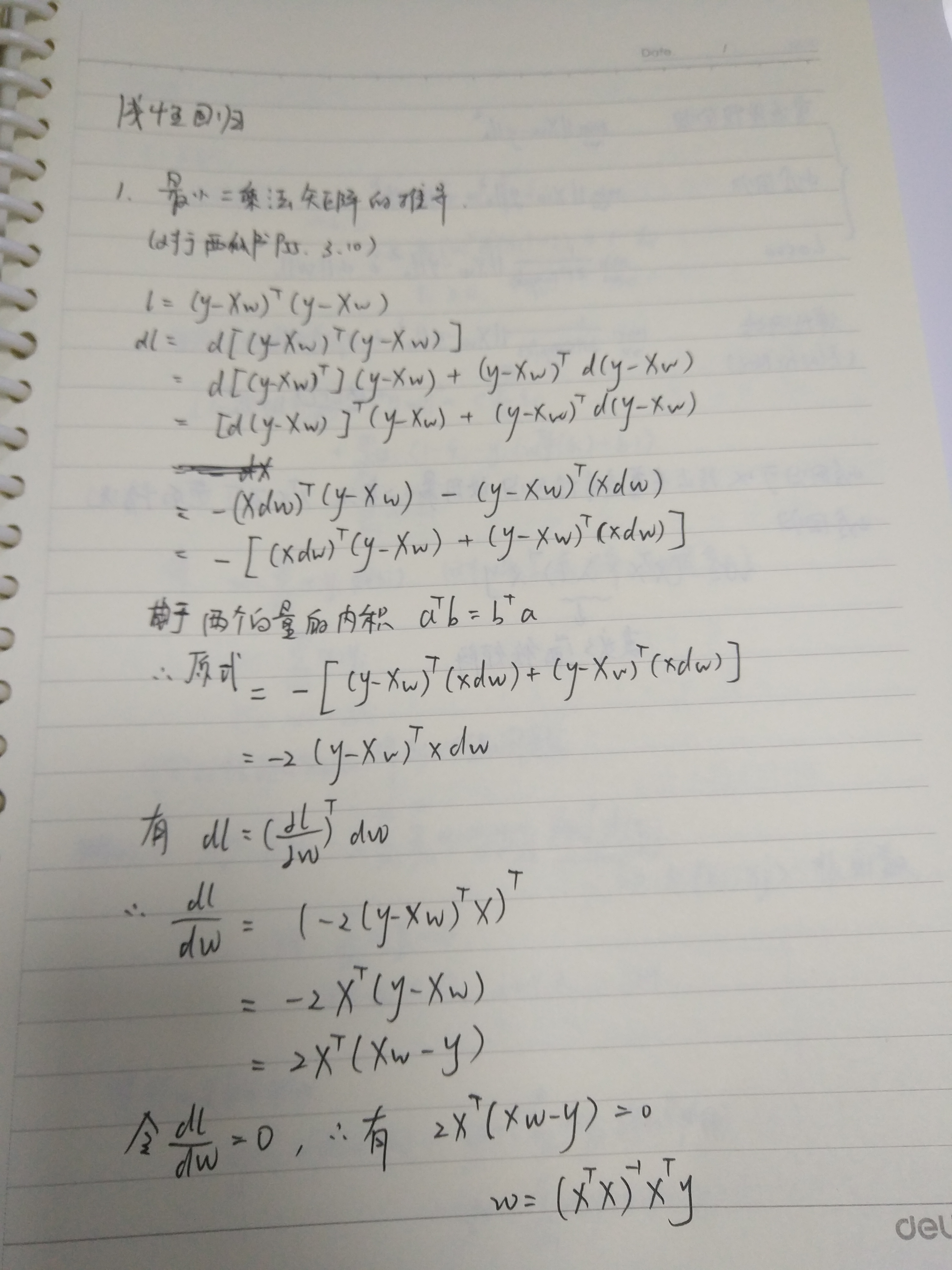梯度下降

$\theta_j:=\theta_j-\alpha\frac{\partial}{\partial\theta_j}J(\theta)$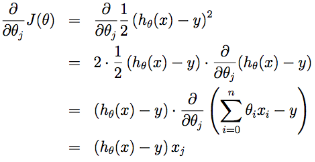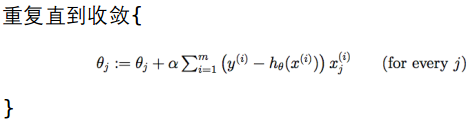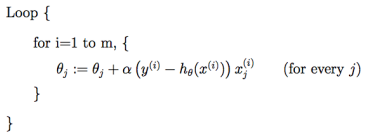SGD相对来说要快很多，但是也有存在问题，由于单个样本的训练可能会带来很多噪声，使得SGD并不是每次迭代都向着整体最优化方向，因此在刚开始训练时可能收敛得很快，但是训练一段时间后就会变得很慢。

岭回归

正规方程

$l=(y-Xw)^T(y-Xw)+\lambda w^Tw$

$w=(X^TX+\lambda I)^{-1}X^Ty$

Lasso

https://www.cnblogs.com/pinard/p/6018889.html

附录

各种误差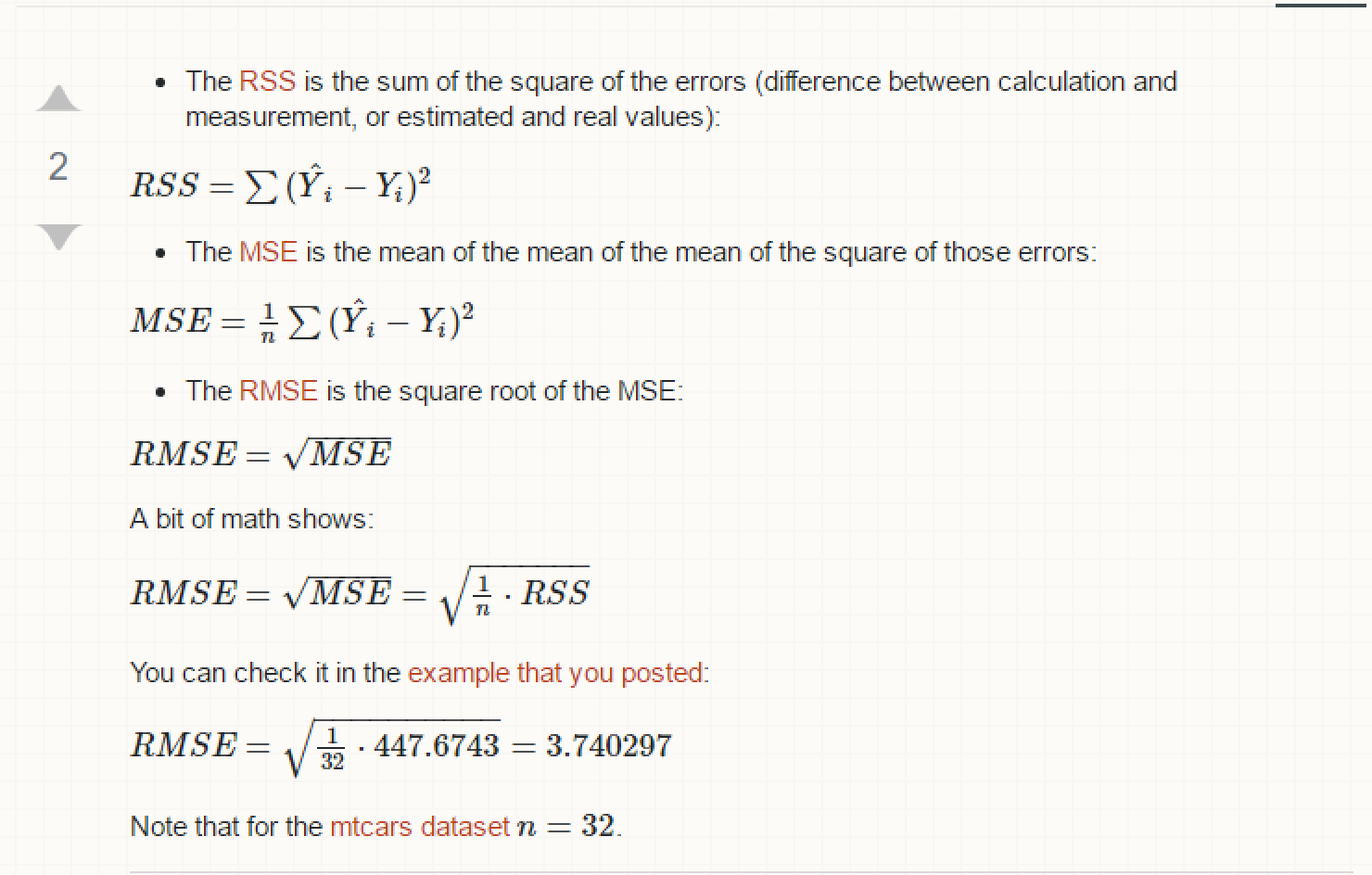MSE(均方误差)、RMSE(均方根误差)、MAE(平均绝对误差)、R-Squared